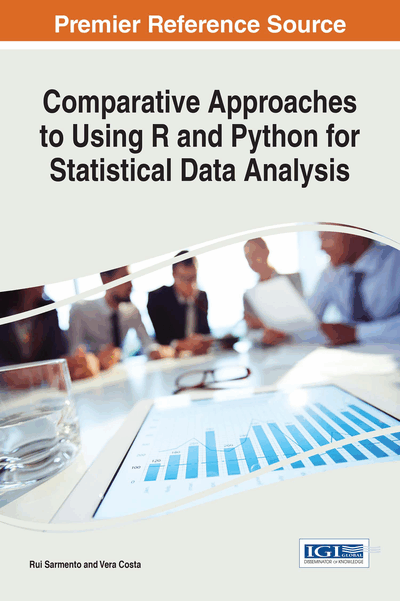# Factor Analysis

Copyright: © 2017 |Pages: 31
DOI: 10.4018/978-1-68318-016-6.ch007
OnDemand PDF Download:
Available
\$37.50
No Current Special Offers

## Abstract

Factor analysis is a statistical method used to describe variability among observed, correlated variables. The goal of performing factor analysis is to search for some unobserved variables called factors. The observed variables are modelled as linear combinations of the possible factors, added the error quantification of this approximation. This added information about the interaction of observed variables could be used for further analysis of the importance of each variable in the context of the dataset. This way, some observed variables are substituted by a set of latent variables in a lower amount, and that, therefore, represents the data in a summarized fashion.
Chapter Preview
Top

## Introduction

Factor analysis is a statistical method used to describe variability among observed, correlated variables. The goal of performing factor analysis is to search for some unobserved variables called factors. This analysis might lead, for example, to the conclusion that it is possible that three unobserved latent variables are reflected in the variations of seven observed variables. The observed variables are modeled as linear combinations of the possible factors, added the error quantification of this approximation. This added information about the interaction of observed variables could be used for further analysis of the importance of each variable in the context of the dataset.

Factor analysis is used in many areas of statistical analysis like, for example, marketing, social sciences, psychology and other situations where a reduction of a large set of variables is adequate to the study being provided. This way, some observed variables are substituted by a set of latent variables in a lower amount, and that, therefore, represent the data in a summarized fashion.

Factor analysis started by being developed before the appearance of modern computers. This beginning of the method was named exploratory factor analysis (EFA). Other variations of factor analysis (for example, confirmatory factor analysis - CFA) will not be explored in this book. Thus, an example of a factorial analysis is presented below.

### Example of a Factorial Analysis

Imagine a Ph.D. Supervisor wants to test the hypothesis there are two kinds of students. A student that “procrastinates” his studies, and the student that does “not procrastinate”, neither of which is an observed variable. Thus, the supervisor only has access to the grades of the student in the several phases a Ph.D. has. Suppose there are ten stages and the student is classified in all those stages. Additionally, the supervisor has a database of 500 Ph.D. students. By choosing each student randomly from this vast universe of students, imagine the grades as being random variables also. The supervisor hypothesis might clarify that for each of the 10 Ph.D. grades, the score averaged over the group of all students who share some common pair of values for procrastination and “not procrastinating” is some constant multiplied by their level of procrastination plus another constant multiplied by their level of low inertia behaviour, i.e., it is a combination of those two “factors”.

The numbers for a particular stage, by which the two kinds of behavior are multiplied to obtain the expected score, are posited by the hypothesis to be the same for all procrastination level pairs and are called “factor loading” for this subject. For example, the assumption may hold that the average student's aptitude in the field of “State-of-the-Art writing” is {11 × the student's “procrastinating”} + {5 × the student's “not procrastinating”}.

The numbers 11 and 5 are the factor loadings associated with the task of writing the State-of-the-Art chapter. Other academic tasks may have different factor loadings.

Two students having similar degrees of procrastination and equal degrees of having low inertia may have different aptitudes in State-of-the-Art writing because individual skills differ from average abilities. That difference is called the “error” - a statistical term that means the amount by which an individual changes from what is average for his or her levels of procrastination.

The observable data that go into factor analysis would be ten stage's scores of each of the 500 students, a total of 5,000 numbers. The factor loadings and levels of the two kinds of inertia of each student should be inferred from the data.

Top

## The Factor Analysis Model

whereare factor values (with),represent thespecific factors andrepresents the weight offactor in the variable(factor loadings), that is, eachmeasures the contribution of thecommon factor in the variable. Without loss of generality, and for convenience,variables can be centered and reduced as. Thus, the factor model can be written by:

## Complete Chapter List

Search this Book:
Reset